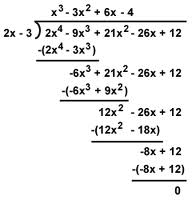# Remainder Theorem & Factor Theorem: Definition & Examples

An error occurred trying to load this video.

Try refreshing the page, or contact customer support.

Coming up next: Dividing Polynomials with Long and Synthetic Division: Practice Problems

### You're on a roll. Keep up the good work!

Replay
Your next lesson will play in 10 seconds
• 0:01 The Division Procedure
• 1:59 The Remainder Theorem
• 3:42 Examples 1 & 2
• 5:14 Factor Theorem
• 5:50 Example 3
• 6:20 Lesson Summary
Save Save

Want to watch this again later?

Timeline
Autoplay
Autoplay
Speed Speed

#### Recommended Lessons and Courses for You

Lesson Transcript
Instructor: David Liano

David has a Master of Business Administration, a BS in Marketing, and a BA in History.

In this lesson, you will learn about the remainder theorem and the factor theorem. You will also learn how to use these theorems to find remainders and factors of polynomials.

## The Division ProcedurePolynomials of degree 3 or higher can sometimes be difficult to factor. Long division and synthetic division are methods that can be used to factor polynomials. These methods are very effective, so you should practice them. However, students always appreciate a shortcut.

This is where the remainder theorem and factor theorem come into play. After completing this lesson, you will know how to use these theorems to find remainders and factors of polynomials. It is important to note, however, that these theorems do not provide as much information as other methods, but the information they provide is sometimes good enough.

Before we introduce the theorems, let's start with a simple problem listing the factors of 27. We could probably easily list the factors as 1, 3, 9, and 27. These numbers are factors because they divide evenly into 27. In other words, if we divide by the factor 3, the remainder is zero. This fact might seem pretty obvious when dividing with integers, but this property is important to understand when dividing with polynomials.

If we divide 27 by some other numbers, we will create a remainder. For instance, if we divide 27 by 7, we will get a remainder of 6.

Finding factors of polynomials, such as quadratic expressions, can be a little more difficult. Well, factoring quadratic expressions is usually not too difficult for the accomplished algebra student. What about finding factors of polynomials that have a degree of 3 or higher? In other words, the lead term has an exponent higher than 2, as in the following example:

x^3 + 2x^2 - 11x - 12

Here we can use long division or synthetic division to find the factors of this polynomial. These methods are very effective. However, the purpose of this lesson is to show alternatives that can be used.

## Remainder Theorem

Let's first state the remainder theorem. The remainder theorem states the following:

If you divide a polynomial f(x) by (x - h), then the remainder is f(h).

The theorem states that our remainder equals f(h). Therefore, we do not need to use long division, but just need to evaluate the polynomial when x = h to find the remainder.

We should understand why this theorem is true. Why does this work?

Let's set up our division problem using the notation in the theorem. If we divide some polynomial f(x) with a divisor in the form of (x - h) of which h is a real number, then we will get a quotient, which we will call p(x), and a remainder, which we will call r. Our division problem will look as follows:

f(x)/(x - h) = p(x) with a remainder of r

Like we did with our simple division problems in integers, we can manipulate this function to get the following.

f(x) = (x - h) * p(x) + r

Let's say we want to evaluate our polynomial at x = h. Therefore, (x - h) = (h - h) = 0. Let's look at the following statements. Statement 1 shows our equation. In statement 2, we substitute h for x. Then we simplify in statements 3 and 4.

1. f(x) = (x - h)p(x) + r (equation)
2. f(h) = (h - h)p(h) + r (substitute h for x)
3. f(h) = (0)p(h) + r (simplify)
4. f(h) = r (simplify)

Do you see what this tells us? As our theorem states, we can find the remainder just by evaluating f(h). We can skip the long division!

## Example 1

f(x) = x^3 + 2x^2 - 11x - 12

Find the remainder if f(x) is divided by x - 2.

To unlock this lesson you must be a Study.com Member.

### Register to view this lesson

Are you a student or a teacher?

#### See for yourself why 30 million people use Study.com

##### Become a Study.com member and start learning now.
Back
What teachers are saying about Study.com

### Earning College Credit

Did you know… We have over 200 college courses that prepare you to earn credit by exam that is accepted by over 1,500 colleges and universities. You can test out of the first two years of college and save thousands off your degree. Anyone can earn credit-by-exam regardless of age or education level.